# Tutorial on Voiced Sound Detection for Singing & Humming Recordings

This tutorial describes the basics of voiced sound detection (VSD). In particular, the audio files used for our analysis are singing/humming recordings with human labeled frame-based pitch as the groundtruth. Here the voiced sound refer to the frames the recordings which have pitch. In other words, this is a binary classification problem given the mixture of vocal and background music. We shall try to tackle this problem with several common methods.

## Preprocessing

Before we start, let's add necessary toolboxes to the search path of MATLAB:

```addpath d:/users/jang/matlab/toolbox/utility
```

All the above toolboxes can be downloaded from the author's toolbox page. Make sure you are using the latest toolboxes to work with this script.

For compatibility, here we list the platform and MATLAB version that we used to run this script:

```fprintf('Platform: %s\n', computer);
fprintf('MATLAB version: %s\n', version);
fprintf('Script starts at %s\n', char(datetime));
scriptStartTime=tic;	% Timing for the whole script
```
```Platform: PCWIN64
MATLAB version: 9.6.0.1214997 (R2019a) Update 6
Script starts at 18-Jan-2020 19:45:18
```

For this script, most of the modifiable options are set in vsdOptSet.m:

```type vsdOptSet
```
```function vsdOpt=vsdOptSet
% vsdOptSet: Set options for VSD (voiced sound detection) for singing/humming
%
%	Usage:
%		vsdOpt=vsdOptSet;
%
%	Description:
%		vsdOpt=vsdOptSet returns the default options for voiced sound detection.
%
%	Example:
%		vsdOpt=vsdOptSet

%	Category: Options for voiced sound detection
%	Roger Jang, 20130114

%% === Basic options for VSD
vsdOpt.fs=16000/2;
vsdOpt.frameSize=512/2;
vsdOpt.overlap=0;
vsdOpt.frameZeroMeanOrder=3;
vsdOpt.gaussianNum=4;	% No. of Gaussians for each class
vsdOpt.outputName={'s/u', 'voiced'};	% s/u: silence & unoviced
vsdOpt.usedChannel=1;	% Use channel 1
%% === Function for feature extraction and plotting
vsdOpt.feaExtractFcn=@hummingFeaExtract;
vsdOpt.hmmPlotFcn=@vsdHmmPlot;
%% === Folder for wave files
vsdOpt.audioDir='\dataset\childSong4public\MIR-QBSH-corpus\waveFile\year2007\person00001';
```

You can change options in this file to try other settings for VSD. In particular, the following changes are mandatory if you want to run this script on your machine:

• Make "vsdOpt.audioDir" point to the audio folder of the MIR-QBSH corpus.

The MIR-QBSH corpus is available at here.

## Dataset collection

We can now read all the audio files (that have been manually labeled with frame-based pitch) to perform feature extraction. Note that: * The result is stored in a big structure varaible auSet for further analysis. * We also save auSet to vsdAuSet.mat so that we don't need to do it again next time. * If the program finds vsdAuSet.mat, it'll load the file directly to restore auSet. * If you prefer to redo the feature extraction, you need to delete "vsdAuSet.mat" in advance.

Here it goes:

```vsdOpt=vsdOptSet;
if ~exist('vsdAuSet.mat', 'file')
myTic=tic;
fprintf('Collecting audio files and their features from "%s"...\n', vsdOpt.audioDir);
auSet=recursiveFileList(vsdOpt.audioDir, 'wav');	% Collect all wav files
auSet=auSetFeaExtract(auSet, vsdOpt);
fprintf('Time for feature extraction over %d files = %g sec\n', length(auSet), toc(myTic));
fprintf('Saving auSet to vsdAuSet.mat...\n');
save vsdAuSet auSet
else
end
```
```Loading auSet from vsdAuSet.mat...
```

Now we can create a variable ds for data visualization and classification:

```ds.input=[auSet.feature];
ds.output=[auSet.tOutput];
ds.inputName=auSet(1).other.inputName;
ds.outputName=vsdOpt.outputName;
```

We can obtain the feature dimensions and data count:

```[dim, count]=size(ds.input);
fprintf('Feature dimensions = %d, data count = %d\n', dim, count);
```
```Feature dimensions = 10, data count = 5000
```

Since the dataset is big, we reduce the dataset for easy plotting:

```downSampleRatio=2;
ds.input=ds.input(:, 1:downSampleRatio:end);
ds.output=ds.output(:, 1:downSampleRatio:end);
fprintf('Data count = %d after 1/%d down sampling.\n', size(ds.input, 2), downSampleRatio);
```
```Data count = 2500 after 1/2 down sampling.
```

## Data analysis and visualization

Display data sizes for each class:

```[classSize, classLabel]=dsClassSize(ds, 1);
```
```10 features
2500 instances
2 classes
```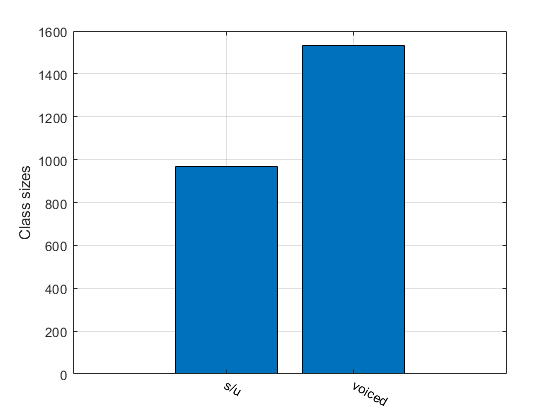Display feature distribution for each class:

```figure; dsBoxPlot(ds);
```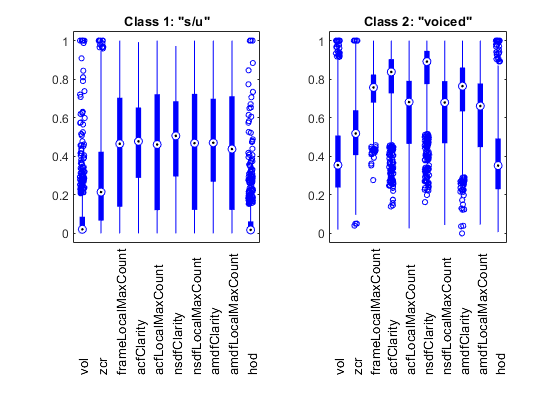Plot the feature vectors within each class:

```figure; dsFeaVecPlot(ds);
```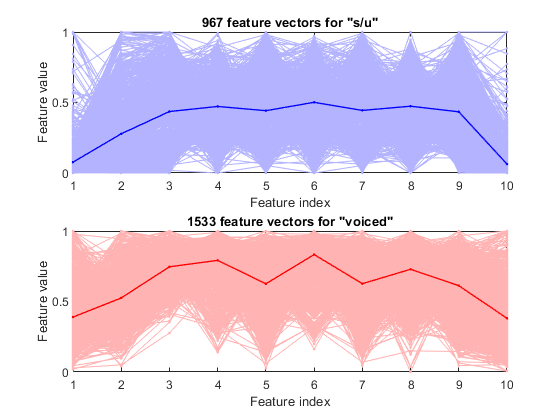Scatter plot of the original ds to 2D:

```figure; dsProjPlot2(ds); figEnlarge
```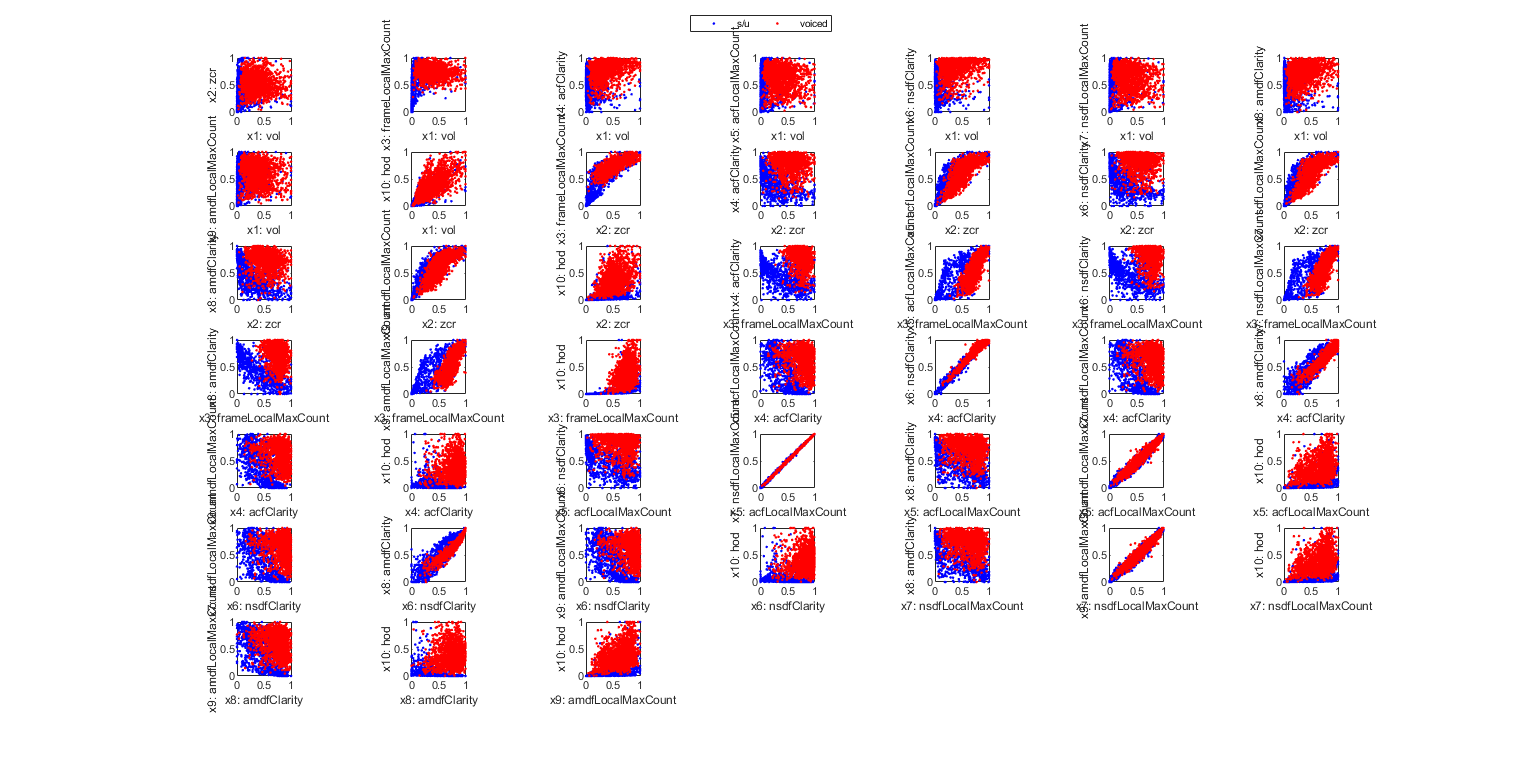Scatter plot of the original ds to 3D:

```figure; dsProjPlot3(ds); figEnlarge
```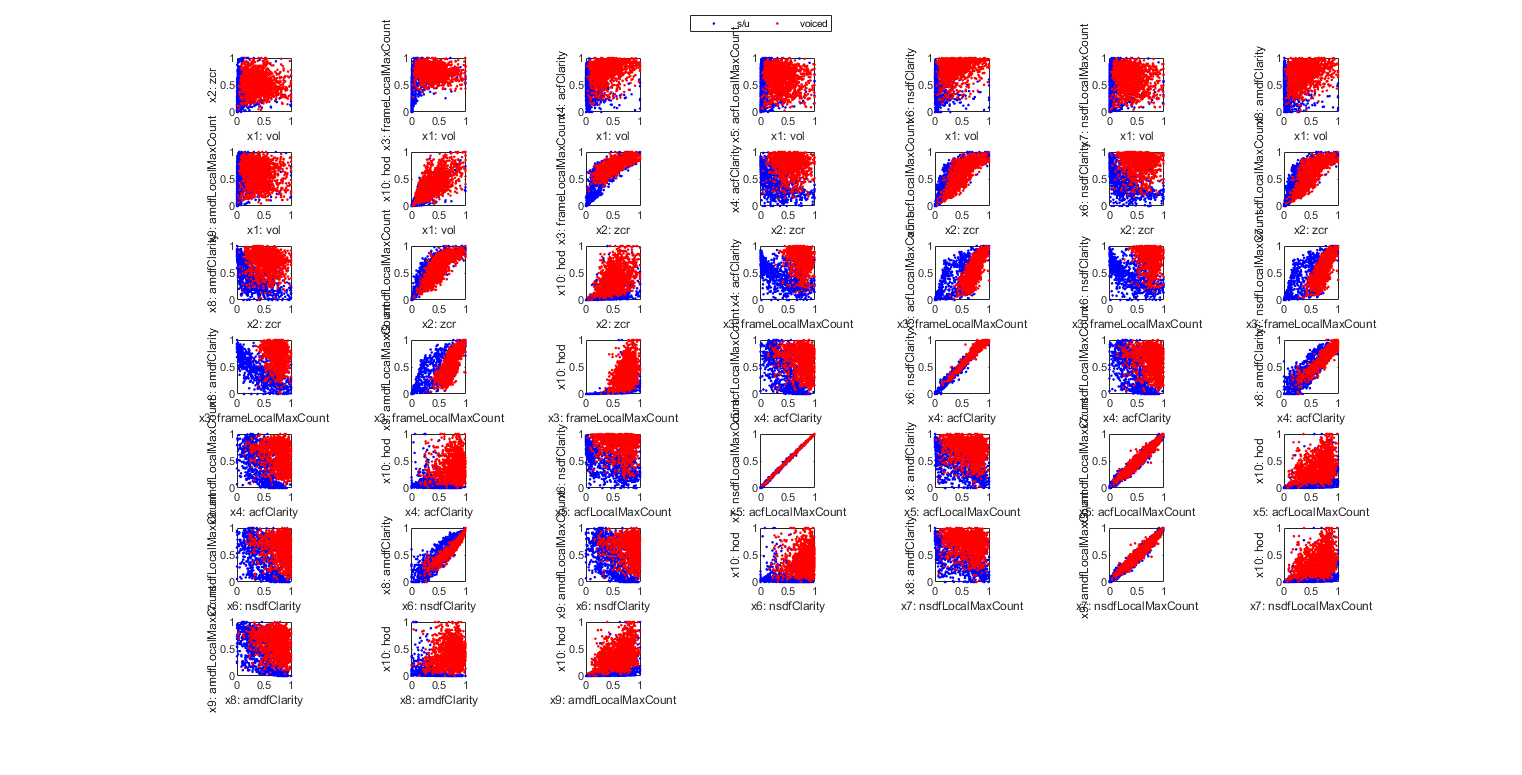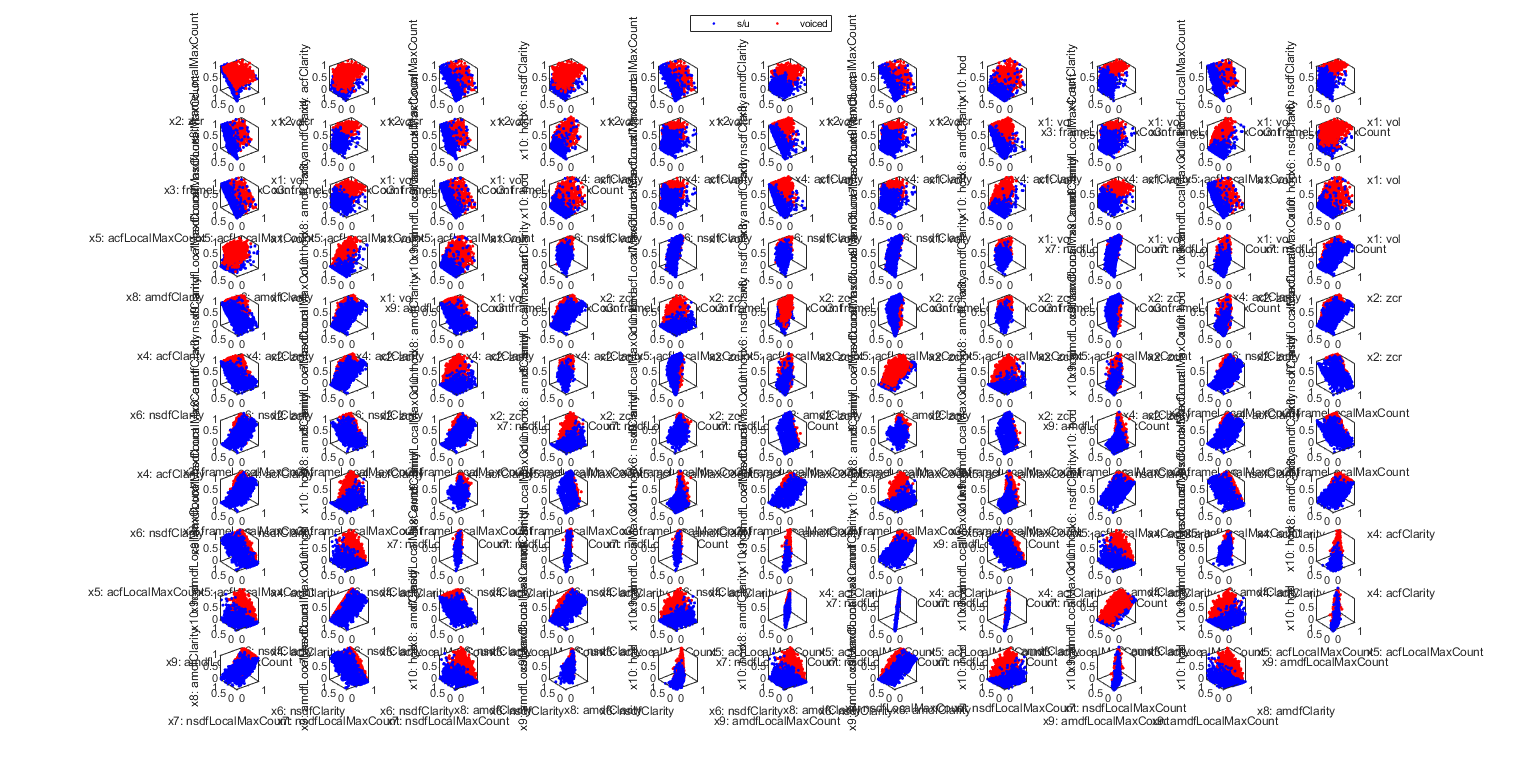## Input selection

We can select more important inputs based on leave-one-out (LOO) criterion of KNNC:

```myTic=tic;
figure; bestInputIndex=inputSelectSequential(ds); figEnlarge;
fprintf('time=%g sec\n', toc(myTic));
```
```Construct 55 "knnc" models, each with up to 10 inputs selected from all 10 inputs...

Selecting input 1:
Model 1/55: selected={vol} => Recog. rate = 84.2%
Model 2/55: selected={zcr} => Recog. rate = 71.6%
Model 3/55: selected={frameLocalMaxCount} => Recog. rate = 71.2%
Model 4/55: selected={acfClarity} => Recog. rate = 73.8%
Model 5/55: selected={acfLocalMaxCount} => Recog. rate = 61.6%
Model 6/55: selected={nsdfClarity} => Recog. rate = 73.5%
Model 7/55: selected={nsdfLocalMaxCount} => Recog. rate = 63.3%
Model 8/55: selected={amdfClarity} => Recog. rate = 66.6%
Model 9/55: selected={amdfLocalMaxCount} => Recog. rate = 61.8%
Model 10/55: selected={hod} => Recog. rate = 85.2%
Currently selected inputs: hod => Recog. rate = 85.2%

Selecting input 2:
Model 11/55: selected={hod, vol} => Recog. rate = 88.1%
Model 12/55: selected={hod, zcr} => Recog. rate = 87.2%
Model 13/55: selected={hod, frameLocalMaxCount} => Recog. rate = 87.3%
Model 14/55: selected={hod, acfClarity} => Recog. rate = 92.0%
Model 15/55: selected={hod, acfLocalMaxCount} => Recog. rate = 87.1%
Model 16/55: selected={hod, nsdfClarity} => Recog. rate = 91.1%
Model 17/55: selected={hod, nsdfLocalMaxCount} => Recog. rate = 88.1%
Model 18/55: selected={hod, amdfClarity} => Recog. rate = 91.5%
Model 19/55: selected={hod, amdfLocalMaxCount} => Recog. rate = 87.1%
Currently selected inputs: hod, acfClarity => Recog. rate = 92.0%

Selecting input 3:
Model 20/55: selected={hod, acfClarity, vol} => Recog. rate = 92.2%
Model 21/55: selected={hod, acfClarity, zcr} => Recog. rate = 91.8%
Model 22/55: selected={hod, acfClarity, frameLocalMaxCount} => Recog. rate = 92.3%
Model 23/55: selected={hod, acfClarity, acfLocalMaxCount} => Recog. rate = 92.4%
Model 24/55: selected={hod, acfClarity, nsdfClarity} => Recog. rate = 92.2%
Model 25/55: selected={hod, acfClarity, nsdfLocalMaxCount} => Recog. rate = 92.6%
Model 26/55: selected={hod, acfClarity, amdfClarity} => Recog. rate = 92.3%
Model 27/55: selected={hod, acfClarity, amdfLocalMaxCount} => Recog. rate = 92.1%
Currently selected inputs: hod, acfClarity, nsdfLocalMaxCount => Recog. rate = 92.6%

Selecting input 4:
Model 28/55: selected={hod, acfClarity, nsdfLocalMaxCount, vol} => Recog. rate = 92.6%
Model 29/55: selected={hod, acfClarity, nsdfLocalMaxCount, zcr} => Recog. rate = 92.3%
Model 30/55: selected={hod, acfClarity, nsdfLocalMaxCount, frameLocalMaxCount} => Recog. rate = 91.8%
Model 31/55: selected={hod, acfClarity, nsdfLocalMaxCount, acfLocalMaxCount} => Recog. rate = 92.2%
Model 32/55: selected={hod, acfClarity, nsdfLocalMaxCount, nsdfClarity} => Recog. rate = 92.2%
Model 33/55: selected={hod, acfClarity, nsdfLocalMaxCount, amdfClarity} => Recog. rate = 92.5%
Model 34/55: selected={hod, acfClarity, nsdfLocalMaxCount, amdfLocalMaxCount} => Recog. rate = 91.8%
Currently selected inputs: hod, acfClarity, nsdfLocalMaxCount, vol => Recog. rate = 92.6%

Selecting input 5:
Model 35/55: selected={hod, acfClarity, nsdfLocalMaxCount, vol, zcr} => Recog. rate = 92.9%
Model 36/55: selected={hod, acfClarity, nsdfLocalMaxCount, vol, frameLocalMaxCount} => Recog. rate = 92.0%
Model 37/55: selected={hod, acfClarity, nsdfLocalMaxCount, vol, acfLocalMaxCount} => Recog. rate = 92.6%
Model 38/55: selected={hod, acfClarity, nsdfLocalMaxCount, vol, nsdfClarity} => Recog. rate = 92.6%
Model 39/55: selected={hod, acfClarity, nsdfLocalMaxCount, vol, amdfClarity} => Recog. rate = 92.3%
Model 40/55: selected={hod, acfClarity, nsdfLocalMaxCount, vol, amdfLocalMaxCount} => Recog. rate = 92.3%
Currently selected inputs: hod, acfClarity, nsdfLocalMaxCount, vol, zcr => Recog. rate = 92.9%

Selecting input 6:
Model 41/55: selected={hod, acfClarity, nsdfLocalMaxCount, vol, zcr, frameLocalMaxCount} => Recog. rate = 92.4%
Model 42/55: selected={hod, acfClarity, nsdfLocalMaxCount, vol, zcr, acfLocalMaxCount} => Recog. rate = 92.6%
Model 43/55: selected={hod, acfClarity, nsdfLocalMaxCount, vol, zcr, nsdfClarity} => Recog. rate = 92.5%
Model 44/55: selected={hod, acfClarity, nsdfLocalMaxCount, vol, zcr, amdfClarity} => Recog. rate = 92.7%
Model 45/55: selected={hod, acfClarity, nsdfLocalMaxCount, vol, zcr, amdfLocalMaxCount} => Recog. rate = 92.8%
Currently selected inputs: hod, acfClarity, nsdfLocalMaxCount, vol, zcr, amdfLocalMaxCount => Recog. rate = 92.8%

Selecting input 7:
Model 46/55: selected={hod, acfClarity, nsdfLocalMaxCount, vol, zcr, amdfLocalMaxCount, frameLocalMaxCount} => Recog. rate = 92.1%
Model 47/55: selected={hod, acfClarity, nsdfLocalMaxCount, vol, zcr, amdfLocalMaxCount, acfLocalMaxCount} => Recog. rate = 92.8%
Model 48/55: selected={hod, acfClarity, nsdfLocalMaxCount, vol, zcr, amdfLocalMaxCount, nsdfClarity} => Recog. rate = 92.7%
Model 49/55: selected={hod, acfClarity, nsdfLocalMaxCount, vol, zcr, amdfLocalMaxCount, amdfClarity} => Recog. rate = 92.8%
Currently selected inputs: hod, acfClarity, nsdfLocalMaxCount, vol, zcr, amdfLocalMaxCount, acfLocalMaxCount => Recog. rate = 92.8%

Selecting input 8:
Model 50/55: selected={hod, acfClarity, nsdfLocalMaxCount, vol, zcr, amdfLocalMaxCount, acfLocalMaxCount, frameLocalMaxCount} => Recog. rate = 92.5%
Model 51/55: selected={hod, acfClarity, nsdfLocalMaxCount, vol, zcr, amdfLocalMaxCount, acfLocalMaxCount, nsdfClarity} => Recog. rate = 92.9%
Model 52/55: selected={hod, acfClarity, nsdfLocalMaxCount, vol, zcr, amdfLocalMaxCount, acfLocalMaxCount, amdfClarity} => Recog. rate = 92.6%
Currently selected inputs: hod, acfClarity, nsdfLocalMaxCount, vol, zcr, amdfLocalMaxCount, acfLocalMaxCount, nsdfClarity => Recog. rate = 92.9%

Selecting input 9:
Model 53/55: selected={hod, acfClarity, nsdfLocalMaxCount, vol, zcr, amdfLocalMaxCount, acfLocalMaxCount, nsdfClarity, frameLocalMaxCount} => Recog. rate = 92.7%
Model 54/55: selected={hod, acfClarity, nsdfLocalMaxCount, vol, zcr, amdfLocalMaxCount, acfLocalMaxCount, nsdfClarity, amdfClarity} => Recog. rate = 92.8%
Currently selected inputs: hod, acfClarity, nsdfLocalMaxCount, vol, zcr, amdfLocalMaxCount, acfLocalMaxCount, nsdfClarity, amdfClarity => Recog. rate = 92.8%

Selecting input 10:
Model 55/55: selected={hod, acfClarity, nsdfLocalMaxCount, vol, zcr, amdfLocalMaxCount, acfLocalMaxCount, nsdfClarity, amdfClarity, frameLocalMaxCount} => Recog. rate = 92.4%
Currently selected inputs: hod, acfClarity, nsdfLocalMaxCount, vol, zcr, amdfLocalMaxCount, acfLocalMaxCount, nsdfClarity, amdfClarity, frameLocalMaxCount => Recog. rate = 92.4%

Overall maximal recognition rate = 92.9%.
Selected 8 inputs (out of 10): hod, acfClarity, nsdfLocalMaxCount, vol, zcr, amdfLocalMaxCount, acfLocalMaxCount, nsdfClarity
time=3.52739 sec
```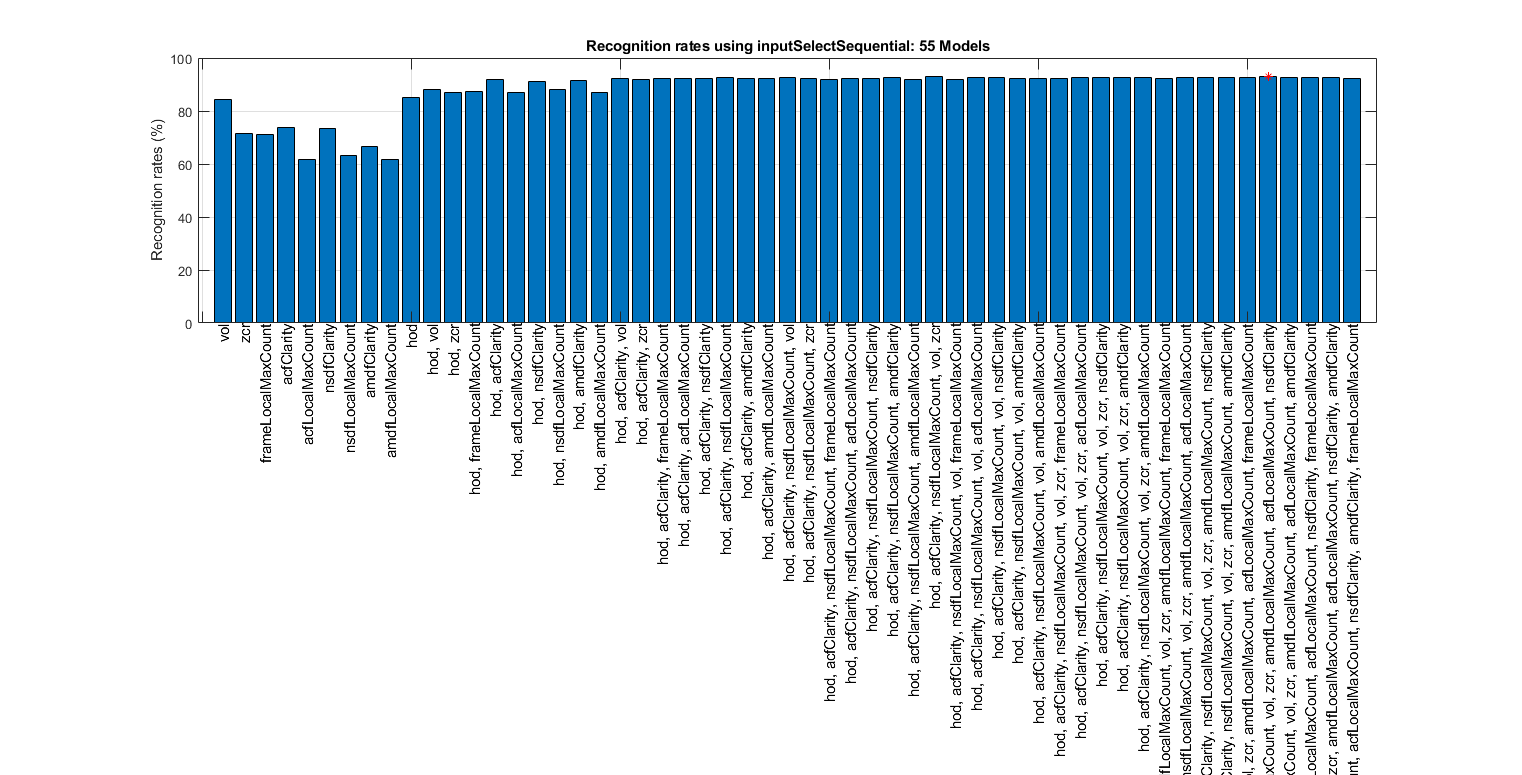## Input transformation

We can also perform LDA and evaluation of its performance based on LOO criterion. To get a quick result, here we find the transformation matrix using all data points and then evaluate KNNC performance based on LOO. This is only an approximate result and it tends to be on the optimistic side:

```myTic=tic;
ds2=ds; ds2.input=inputNormalize(ds2.input);	% input normalization
opt=ldaPerfViaKnncLoo('defaultOpt');
opt.mode='approximate';
recogRate1=ldaPerfViaKnncLoo(ds, opt);
recogRate2=ldaPerfViaKnncLoo(ds2, opt);
figure; plot(1:length(recogRate1), 100*recogRate1, 'o-', 1:length(recogRate2), 100*recogRate2, '^-'); grid on
legend('Raw data', 'Normalized data', 'location', 'northOutside', 'orientation', 'horizontal');
xlabel('No. of projected features based on LDA');
ylabel('LOO recognition rates using KNNC (%)');
fprintf('time=%g sec\n', toc(myTic));
```
```time=1.54574 sec
```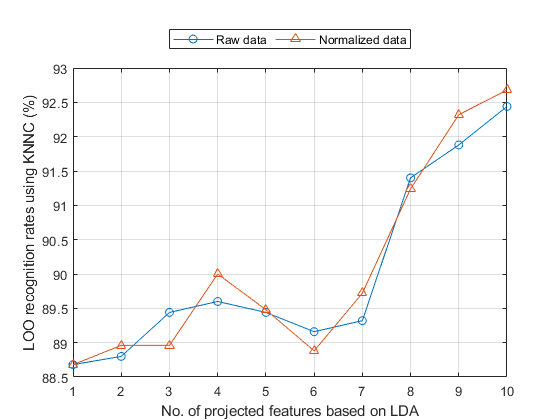We can also perform the LDA evaluation of exact LOO, which is time consuming:

```myTic=tic;
ds2=ds; ds2.input=inputNormalize(ds2.input);	% input normalization
opt=ldaPerfViaKnncLoo('defaultOpt');
opt.mode='exact';
recogRate1=ldaPerfViaKnncLoo(ds, opt);
recogRate2=ldaPerfViaKnncLoo(ds2, opt);
figure; plot(1:length(recogRate1), 100*recogRate1, 'o-', 1:length(recogRate2), 100*recogRate2, '^-'); grid on
legend('Raw data', 'Normalized data', 'location', 'northOutside', 'orientation', 'horizontal');
xlabel('No. of projected features based on LDA');
ylabel('LOO recognition rates using KNNC (%)');
fprintf('time=%g sec\n', toc(myTic));
```
```time=51.0133 sec
```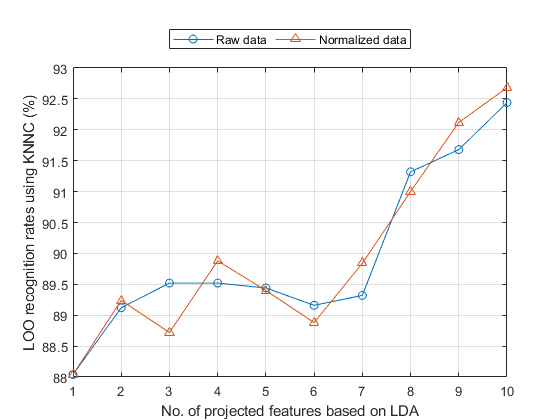## HMM training

Using the collected auSet, we can start HMM training for voiced sound detection:

```myTic=tic;
[vsdHmmModel, rr]=hmmTrain4audio(auSet, vsdOpt, 1); figEnlarge;
fprintf('Time for HMM training=%g sec\n', toc(myTic));
```
```Time for HMM training=2.54971 sec
```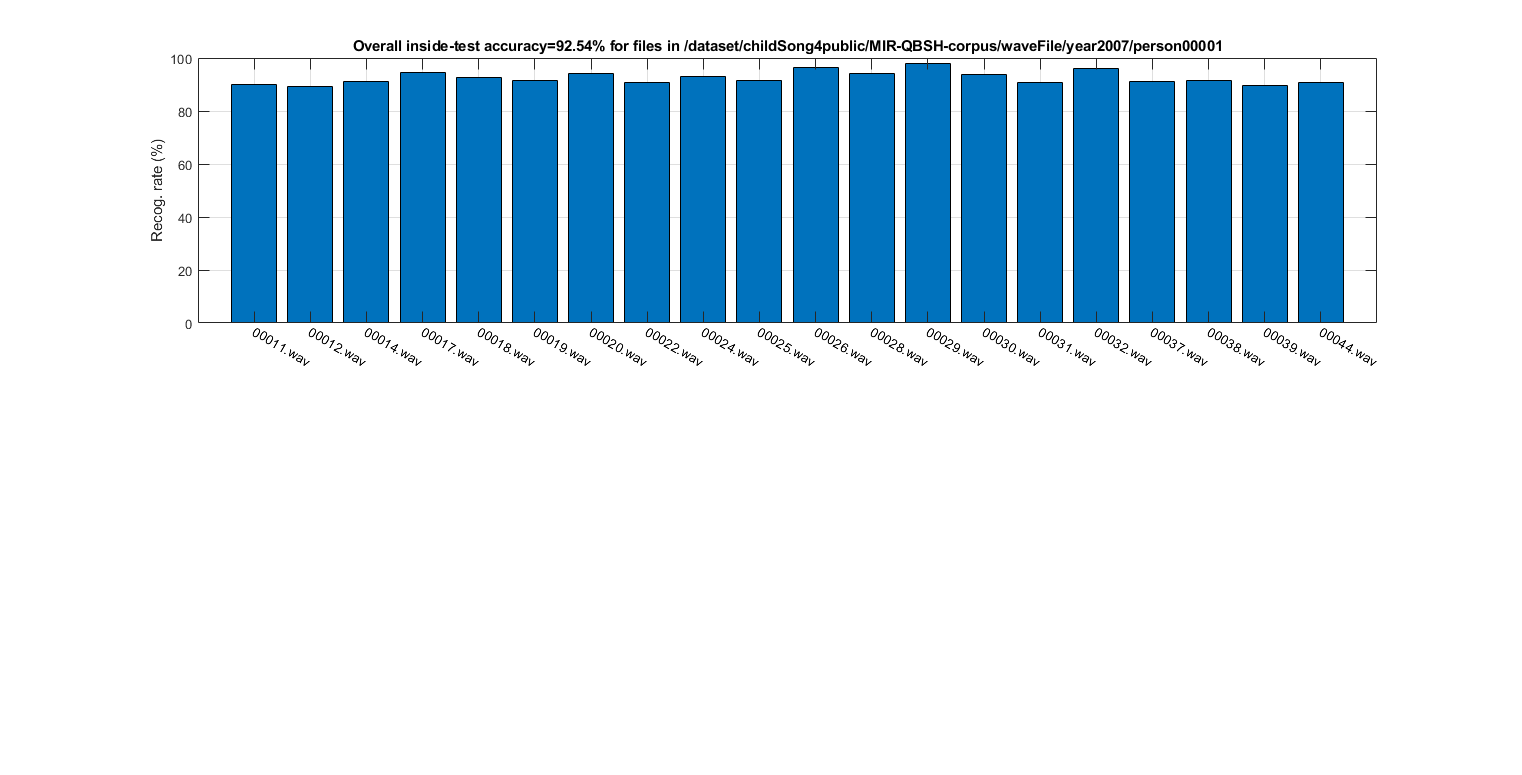## HMM test

After the training, we can test the HMM using a single audio file:

```myTic=tic;
auFile=auSet(1).path;
au=vsdOpt.feaExtractFcn(au, vsdOpt, 1);	% Attach feature and tOutput
wObj=hmmEval4audio(au, vsdOpt, vsdHmmModel, 1); figEnlarge;
fprintf('time=%g sec\n', toc(myTic));
```
```Dot indexing is not supported for variables of this type.

Error in frame2clarity (line 49)
index1=round(fs/pitch2freq(opt.maxPitch));

Error in hummingFeaExtract (line 58)
[acfClarity, acfMat]=frame2clarity(frameMat, au.fs, 'acf');

Error in goTutorial (line 130)
au=vsdOpt.feaExtractFcn(au, vsdOpt, 1);	% Attach feature and tOutput
```

## Performance evaluation of HMM via LOO

To evaluate the performance more objectively, we can test the LOO accuracy by using "leave-one-file-out". (Note that it's very time consuming.)

```myTic=tic;
showPlot=1;
[outsideRr, cvData]=hmmPerfLoo4audio(auSet, vsdOpt, showPlot); figEnlarge;
fprintf('time=%g sec\n', toc(myTic));
```

Now we shall try HMM with the inputs selected previously to see if less inputs can improve the performance. First let's list the selected inputs:

```fprintf('Selected inputs = %s\n', cell2str(ds.inputName(bestInputIndex)));
```
```myTic=tic;
% The we can use the selected inputs for HMM training/test:
for i=1:length(auSet)
auSet(i).feature=auSet(i).feature(bestInputIndex,:);
end
[outsideRr, cvData]=hmmPerfLoo4audio(auSet, vsdOpt, 1);
fprintf('time=%g sec\n', toc(myTic));
```

It turns out that the HMM using the 4 selected inputs outperforms the HMM using all inputs.

We can then detect the best number of Gaussian components in each state of the HMM using such leave-one-file-out cross validation. This part is also time consuming:

```myTic=tic;
maxExponent=6;
insideRr=zeros(1, maxExponent);
for i=1:maxExponent
vsdOpt.gaussianNum=2^i;
fprintf('%d/%d: No. of Gaussian component=%d, ', i, maxExponent, vsdOpt.gaussianNum);
[outsideRr(i), cvData]=hmmPerfLoo4audio(auSet, vsdOpt);
insideRr(i)=mean([cvData.insideRr]);
fprintf('insideRR=%g%%, outsideRr=%g%%\n', insideRr(i)*100, outsideRr(i)*100);
end
plot(1:maxExponent, insideRr*100, '.-', 1:maxExponent, outsideRr*100, '.-'); grid on; figEnlarge;
set(gca, 'xTick', 1:maxExponent);
label=get(gca, 'xticklabel');
for i=1:length(label), label{i}=sprintf('2^%s', label{i}); end;
set(gca, 'xticklabel', label);
legend('Inside-test RR', 'Outside-test RR', 'location', 'northOutside', 'orientation', 'horizontal');
xlabel('No. of Gaussian mixtures');
ylabel('Recognition rate (%)');
fprintf('time=%g sec\n', toc(myTic));
```

## Summary

This is a brief tutorial on using HMM for voiced sound detection for QBSH queries. There are several directions for further improvement:

• Investigate new features for VSD.
• Change the configuration of the GMM used in HMM.
• Use of other classifiers for VSD.

## Appendix

List of functions and datasets used in this script

Date and time when finishing this script:

```fprintf('Date & time: %s\n', char(datetime));
```

Overall elapsed time:

```toc(scriptStartTime)
```

Date and time when finishing this script:

```fprintf('Date & time: %s\n', char(datetime));
```